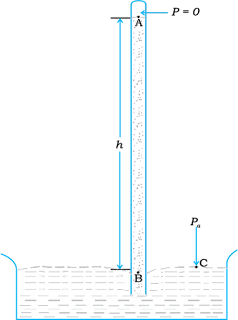# Atmospheric Pressure and Gauge Pressure

The pressure of the atmosphere at any point is equal to the weight of a column of air of unit cross-sectional area extending from that point to the top of the atmosphere. At sea level, it is 1.013 × 10$^5$  Pa (1 atm). Italian scientist Evangelista Torricelli (1608–1647) devised for the first time a method for measuring atmospheric pressure.Fig 1 The mercury barometer.

A long glass tube closed at one end and filled with mercury is inverted into a trough of mercury as shown in Fig.1.

This device is known as ‘mercury barometer’. The space above the mercury column in the tube contains only mercury vapour whose pressure P is so small that it may be neglected. Thus, the pressure at Point A = 0. The pressure inside the coloumn at Point B must be the same as the pressure at Point C, which is atmospheric pressure, P$_a$.

P$_a$  = ρgh      (1)

where ρ is the density of mercury and h is the height of the mercury column in the tube.

In the experiment it is found that the mercury column in the barometer has a height of about 76 cm at sea level equivalent to one atmosphere (1 atm). This can also be obtained using the value of ρ in Eq. (1). A common way of stating pressure is in terms of cm or mm of mercury (Hg). A pressure equivalent of 1 mm is called a torr (after Torricelli).

1 torr = 133 Pa.

The mm of Hg and torr are used in medicine and physiology. In meteorology, a common unit is the bar and millibar.

1 bar = 105 PaFig 2 The open tube manometer

An open tube manometer is a useful instrument for measuring pressure differences. It consists of a U-tube containing a suitable liquid i.e., a low density liquid (such as oil) for measuring small pressure differences and a high density liquid (such as mercury) for large pressure differences. One end of the tube is open to the atmosphere and the other end is connected to the system whose pressure we want to measure [see Fig. 2]. The pressure P at A is equal to pressure at point B. What we normally measure is the gauge pressure, which is P − P$_a$ , given by Eq. (1) and is proportional to manometer height h.

Pressure is same at the same level on both sides of the U-tube containing a fluid. For liquids, the density varies very little over wide ranges in pressure and temperature and we can treat it safely as a constant for our present purposes. Gases on the other hand, exhibits large variations of densities with changes in pressure and temperature. Unlike gases, liquids are, therefore, largely treated as incompressible.

Example 1

The density of the atmosphere at sea level is 1.29 kg/m$^3$ . Assume that it does not change with altitude. Then how high would the atmosphere extend?

We use Eq. (3)

ρgh = 1.29 kg.m$^{–3}$ × 9.8 m.s$^2$ × h m = 1.01 × 10$^5$  Pa

∴ h = 7989 m ≈ 8 km

In reality the density of air decreases with height. So does the value of g. The atmospheric cover extends with decreasing pressure over 100 km. We should also note that the sea level atmospheric pressure is not always 760 mm of Hg. A drop in the Hg level by 10 mm or more is a sign of an approaching storm.

Example 2

At a depth of 1000 m in an ocean
(a) what is the absolute pressure?
(b) What is the gauge pressure?
(c) Find the force acting on the window of area 20 cm × 20 cm of a submarine at this depth, the interior of which is maintained at sea-level atmospheric pressure.

(The density of sea water is 1.03 × 10$^3$  kg.m$^{-3}$, g = 10 m.s$^{–2}$.)

Here h = 1000 m and ρ = 1.03 × 10$^3$  kg.m$^{-3}$ .

(a) From Eq. (2), absolute pressure

P = P$_a$  + ρgh

= 1.01 × 10$^5$  Pa + 1.03 × 10$^3$ kg.m$^{–3}$ × 10 m.s$^{–2}$ × 1000 m

= 104.01 × 10$^5$  Pa

≈ 104 atm

(b) Gauge pressure is

P − P$_a$  = ρgh = P$_g$

P$_g$  = 1.03 × 10$^3$  kg.m$^{–3}$ × 10 m.s$^2$ × 1000 m

= 103 × 10$^5$  Pa

≈ 103 atm

(c) The pressure outside the submarine is P = P$_a$  + ρgh and the pressure inside it is Pa . Hence, the net pressure acting on the window is gauge pressure, P$_g$  = ρgh.

Since the area of the window is A = 0.04 m$^2$ , the force acting on it is

F = P$_g$ A

= 103 × 10$^5$ Pa × 0.04 m$^2$

F = 4.12 × 10$^5$  N